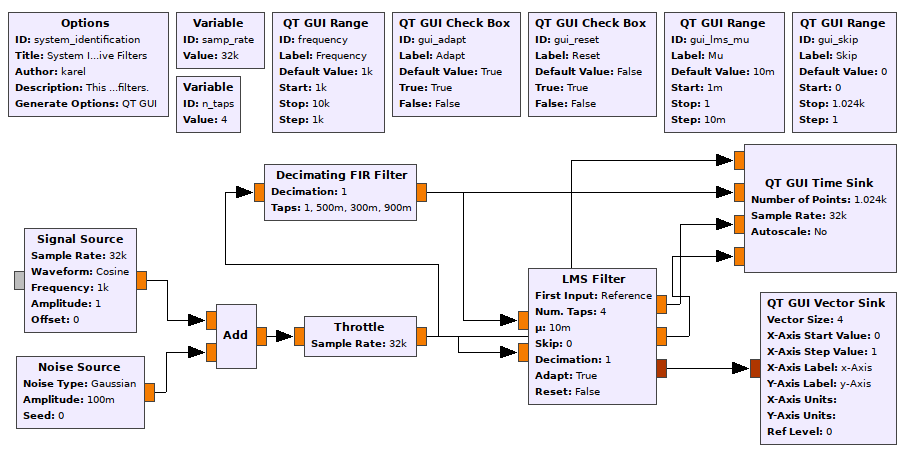# Estimate a microphone's transfer function

I am trying to compare the transfer functions of k different microphones and need some advice on my current approach. As of now, I have attempted the following:

1. Play a pink noise test tone $(X)$ from a speaker and record the tone on all $k$ target microphones. Let's call the outputs as $Y_{i}$.

2. Compute the inverse pink noise tone and convolve it with the recorded microphone tones $Y_{i}$ separately. The output of this convolution operation should be the estimated transfer function ($H_{i}$) of each microphone (it will not be 100% accurate because there is an effect of the room conditions, but let's ignore it for now).

Now to validate that the estimated transfer functions of all microphones are correct, I plan to compute the inverse transfer function $(H^{-1})$, multiply it with each microphone output $Y_i$ in the frequency domain and recover the input signal X. Finally, if all goes well, I expect the recovered X to be the same for all microphones.

$$Y_i = H_i . X$$

$$X = H_i^{-1} . Y_i$$

Are there any other approaches to do this? Any pointers for Python implementations of it?

• Does the speaker you're playing pink noise from have a flat frequency response? (Probably not) Is the room anechoic? Aug 3 '18 at 18:57

Your $H^{-1}$ is effectively what is called a zero-forcing equalizer for the channel represented by $H$. That channel incorporates DAC, amplifier, speaker, your room, the microphone, amplifier, ADC and several filters necessary to fulfill sampling conditions.

Problem is that for any frequency where $H$ has a notch, $H^{-1}$ has to have a very strong amplification! Sadly, that means that the frequencies where your estimate is based on the least received energy have the largest amplitude in the resulting inverted channel model. That leads to amplified noise.

There's a lot of ways to estimate a channel inverse; the MMSE estimator is pretty popular in communications.

Because you ask for a Python implementation: GNU Radio is usually used from Python, and there's multiple equalizers that come with it (try first!); and, there's modules like gr-adapt which allow for calculating (and using!) channel-reversing filters adaptively. What I especially like about gr-adapt is that its documentation and comments are plentiful and come with references.

For example, have a look at https://github.com/karel/gr-adapt/tree/master/examples :Here, gr-adapt's LMS Filter is used to estimate (and invert) the channel (which is simulated using the Decimating FIR Filter block).

• I provided my Matlab code for an LMS equalizer here: dsp.stackexchange.com/questions/31318/…. Here I used it to determine the channel between Left and Right speakers with good results. Aug 3 '18 at 22:20